Describing Variation

Fri 22 November 2013

The 3rd in a series of tutorials on using Python for introductory statistical analysis, this tutorial covers methods for describing data via simple statistical calculations and statistical graphics. As always, the notebook for this tutorial is available here.

In the 1880s, Sir Francis Galton, one of the pioneers of statistics, collected data on the heights of approximately 900 adult children and their parents in London. Galton was interested in studying the relationship between a full-grown child’s height and his or her mother’s and father’s height. In order to do so, Galton collected height measurements from about 200 families in the city of London.

As a setting to illustrate computer techniques for describing variability, take the data that Galton collected on the heights of adult children and their parents. The file "galton.csv" stores these data in a modern, case/variable format.

[galton.csv][link]

In :
import numpy as np
import pandas as pd

Simple Statistical Calculations¶

Simple numerical descriptions are easy to compute. Here are the mean, median, standard deviation and variance of the children’s heights (in inches).

In :
gal.height.mean()
Out:
66.760690423162515
In :
gal.height.median()
Out:
66.5
In :
gal.height.std()
Out:
3.5829184699744614
In :
gal.height.var()
Out:
12.837304762484134

Notice that the variance function (var()) returns the square of the standard deviation (std()). Having both is merely a convenience. A percentile tells where a given value falls in a distribution. For example, a height of 63 inches is on the short side in Galton’s data:

In :
import scipy.stats as st # import some useful stats function from the scipy.stats library
st.percentileofscore(gal.height.values, 63, kind="weak")
Out:
19.153674832962139

Note that in the above case, kind=”weak” corresponds to the definition of a ‘cumulative distribution function’. A percentileofscore() of 80% means that 80% of values are less than or equal to the provided score. This usage is consistent with how the ‘mosaic’ R package calculates percentiles, using the pdata function.

Only about 19% of the cases have a height less than or equal to 63 inches. The percentileofscore() function from the scipy.stats package takes an array (values in a column) as a first argument and finds where the ‘score’ (second argument) falls in the distribution of values in the array. We use the values attribute of the height column from the gal data frame to get the values in the column as an array.

A quantile refers to the same sort of calculation, but inverted. Instead of giving a value in the same units as the distribution, you give a percentage: a number between 0 and 100. The scoreatpercentile() function then calculates the value whose percentile would be that value:

In :
st.scoreatpercentile(gal.height.values, per=20)
Out:
63.5

Note that numpy has a simpler version of this function, but it uses a different naming convention which readers of ‘Statistical Modeling: A Fresh Approach’ and these tutorials might find confusing. In this case, the function is called percentile, and returns the ‘score’ or value at a given percentile. For example, np.percentile(gal.height, 20) returns 63.5 as in the above example.

You can also use the following functionality from ‘pandas’ which is consistent with how the ‘mosaic’ R package calculates quantiles, using the qdata function, and is a bit simpler. Note that instead of a percentage, a probability is given (a number between 0 and 1), but the output is the same.

In :
gal.height.quantile(0.2)
Out:
63.5

Building on this, the 25th and 75th percentiles - in other words, the 50 percent coverage interval, can be computed as:

In :
gal.height.quantile(.25), gal.height.quantile(.75)
Out:
(64.0, 69.700000000000003)

The 50 percent coverage interval can also be computed as a single command using ‘list comprehension’ - a ‘Pythonic’ way to clearly and concisely construct lists.

In :
[gal.height.quantile(q) for q in [.25, .75]]
Out:
[64.0, 69.700000000000003]

[List comprehensions][lists] can be used to construct lists in a very natural, easy way, like a mathematician is used to do. The following are common ways to describe lists (or sets, or tuples, or vectors) in mathematics: $S = \{ x^{2} : \{x \in 0 \dots 9\} \}$ $V = (1, 2, 4, 8, \dots, 2^{12})$ $M = \{x$ $|$ $x$ $\in S$ and $x$ even$\}$ You probably know things like the above from previous math courses. In Python, you can write these expression almost exactly like a mathematician would do, without having to remember any special cryptic syntax. This is how you do the above in Python: S = [x**2 for x in range(10)] V = [2**i for i in range(13)] M = [x for x in S if x % 2 == 0]

We can use the same techniques to compute the 2.5th and 97.5th percentiles - in other words, the 95 percent coverage interval:

In :
[gal.height.quantile(q) for q in [.025, .975]]
Out:
[60.0, 73.0]

Another simple way to compute different coverage intervals is to provide a percentile_width argument to the describe() function:

In :
gal.height.describe(percentile_width=50)[[4,6]] # Subset to return only the coverage interval values
Out:
25%    64.0
75%    69.7
Name: height, dtype: float64

The interquartile range is the width of the 50 percent coverage interval: the diﬀerence between the 75th and 25th percentiles:

In :
np.diff([gal.height.quantile(q) for q in [.25, .75]])
Out:
array([ 5.7])

Simple Statistical Graphics¶

There are several basic types of statistical graphics to display the distribution of a variable: histograms, density plots, and boxplots. These work in a manner that’s similar to mean(), quantile() and so on in terms of syntax, but there are a few important additional items to consider. Firstly, most plotting functions we use will come from the ‘matplotlib’ Python library, which integrates nicely with numpy and other Scientific Python libraries. There are several different ways that we can interact with ‘matplotlib’, inlcuding via the pyplot interface (which is simply a submodule of ‘matplotlib’ and is useful for ‘scripting’) and the pylab interface, which is useful for interactive plotting.

If you prefer to use the pyplot interface, then remember to import it in the usual way at the beginning of your Python session: import matplotlib.pyplot

If you want interactive plotting (and why wouldn’t you?!), start ‘IPython’ with the --pylab flag. With this flag enabled, you don’t have to import matplotlib, as it will be done for you automatically):

ipython --pylab

Or, for inline figures in an IPython qtconsole or notebook, use:

ipython notebook --pylab inline

</span>

Histograms and Distributions¶

Constructing a histogram involves dividing the range of a variable up into bins and counting how many cases fall into each bin. This is done in an almost entirely automatic way using the hist() function from a column in a dataframe:

In :
h = gal.height.hist()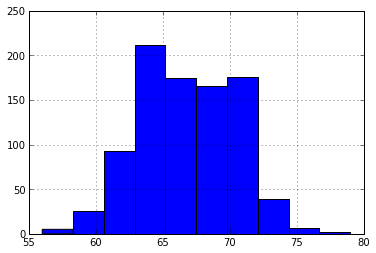You can also create a histogram using ‘pylab’ commands directly:

In :
h = hist(gal.height.values)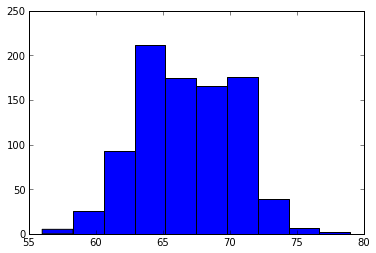When constructing a histogram, Python makes an automatic but sensible choice of the number of bins. If you like, you can control this yourself. For instance:

In :
h = gal.height.hist(bins=25)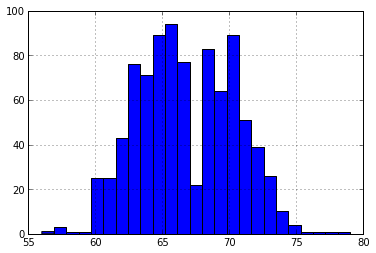The horizontal axis of the histogram is always in the units of the variable. For the histograms above, the horizontal axis is in “inches” because that is the unit of the height variable in the galton dataset. The vertical axis is conventionally drawn in one of two ways, controlled by an optional argument named normed:

• Absolute Frequency or Counts - A simple count of the number of cases that falls into each bin. This is the default, as in:
gal.height.hist()
• Normalized - The vertical axis area of the bar gives the relative proportion of cases that fall into the bin. In other words, the areas can be interpreted as probabilities and the area under the entire histogram is equal to 1. Set the normed argument to True, as in:
gal.height.hist(normed=True)

You can also produce a histogram of relative frequencies, where the vertical axis is scaled so that the height of the bar give the proportion of cases that fall into the bin:

gal.height.hist(weights=np.zeros_like(gal.height) + 100. / len(gal.height))

Other useful optional ‘pylab’ commands set the labels for the axes and the graph as a whole and color the bars. For example,

In :
xlabel("Height (inches)")
ylabel("Density")
title("Distribution of Heights")
grid()
h = gal.height.hist(normed=True, color="grey")
show()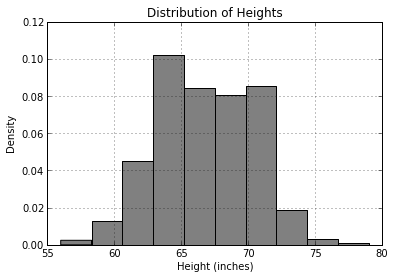The above plot requires multiple lines of commands to produce. Python evaluates each line on its own, updating the plot as each command is issued. Once the histogram is created, we can ‘show’ it with show(). Notice also the use of quotation marks to delimit the labels and names like “grey”.

Density Plots¶

A density plot avoids the need to create bins and plots out the distribution as a continuous curve. Making a density plot generally involves two operations. First, a density function performs the basic density computation, which is then displayed using the plot() function. Pandas provides a shorthand for these two operations to produce a simple ‘density plot’ (where kind="kde" stands for “kind of plot equals ‘kernel density estimator’”):

In :
d = gal.height.plot(kind="kde")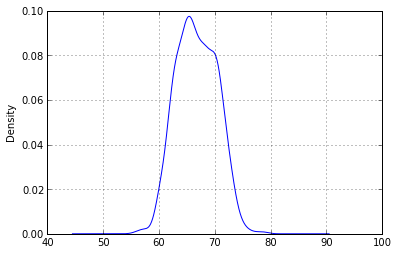Box-and-Whisker Plots¶

Box-and-whisker plots are made with the boxplot() command:

In :
b = gal.boxplot(column="height")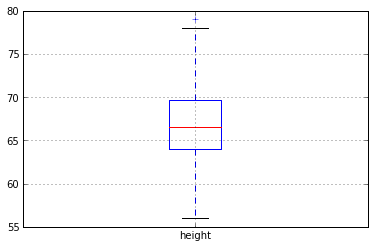The median is represented by the red line in the middle. Outliers, if any, are marked by xs outside the whiskers.

Note that instead of using gal.height, the boxplot() function operatres on the data frame, and takes the column name via the column argument. This is because the real power of the box-and-whisker plot is in comparing distributions. This will be raised again more systematically in later tutorials, but just to illustrate, here is how to compare the heights of males and females:

In :
b = gal.boxplot(column="height", by="sex")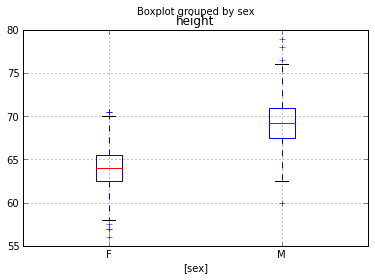Displays of Categorical Variables¶

For categorical variables, it makes no sense to compute descriptive statistics such as the mean, standard deviation, or variance. Instead, look at the number of cases at each level of the variable.

In :
gal.sex.value_counts()
Out:
M    465
F    433
dtype: int64

Proportions can be found in a similar way (use float() to return a ‘float’ rather than an ‘integer’):

In :
gal.sex.value_counts()/float(gal.sex.count())
Out:
M    0.517817
F    0.482183
dtype: float64

Reference¶

As with all ‘Statistical Modeling: A Fresh Approach for Python’ tutorials, this tutorial is based directly on material from ‘Statistical Modeling: A Fresh Approach (2nd Edition)’ by Daniel Kaplan. This tutorial is based on Chapter 3: Describing Variation.

I have made an effort to keep the text and explanations consistent between the original (R-based) version and the Python tutorials, in order to keep things comparable. With that in mind, any errors, omissions, and/or differences between the two versions are mine, and any questions, comments, and/or concerns should be directed to me.